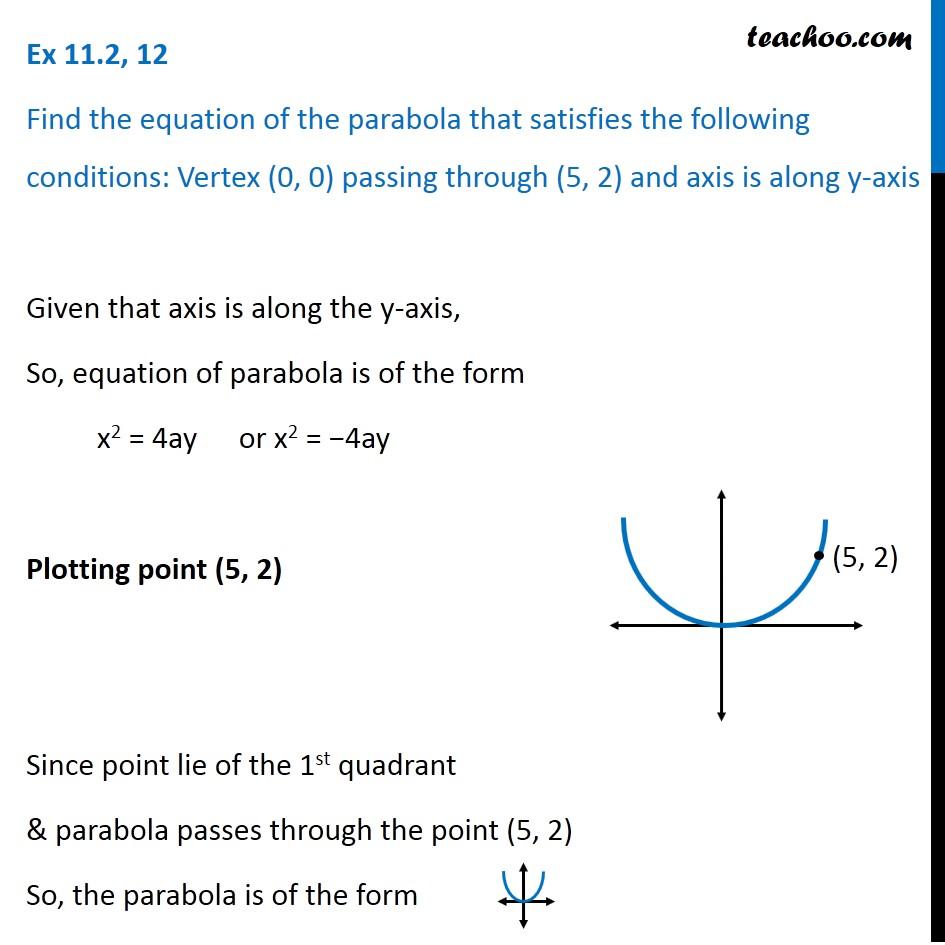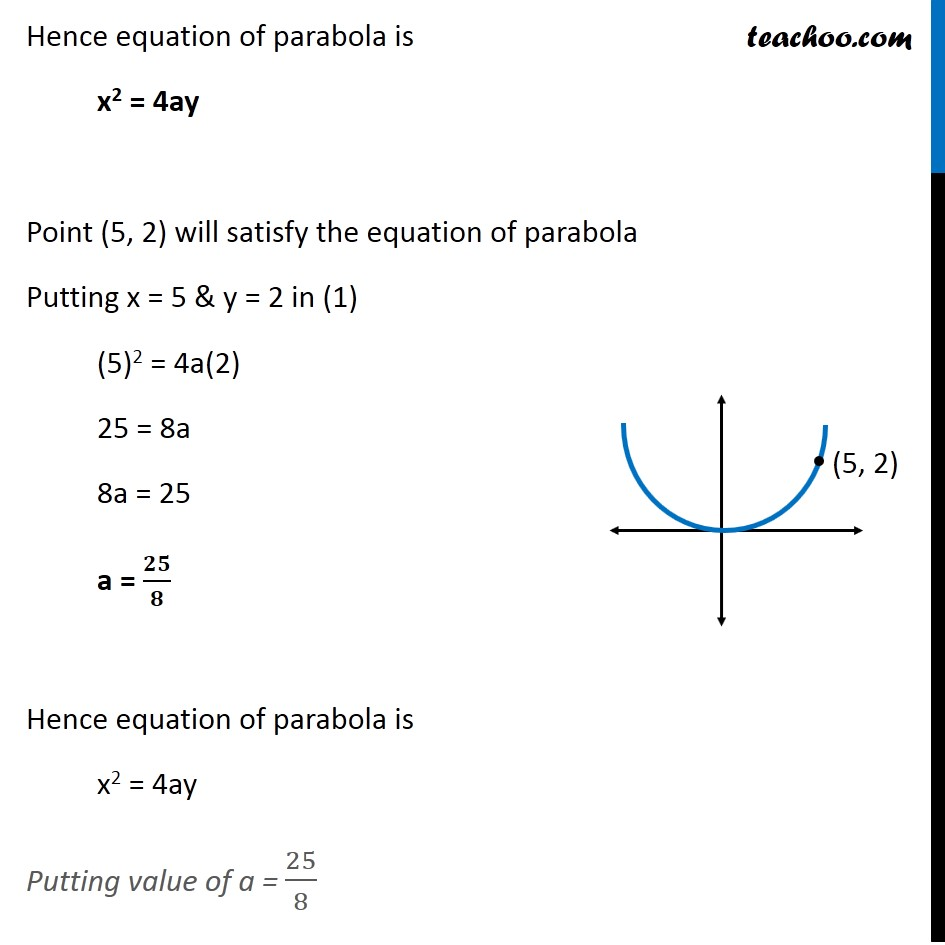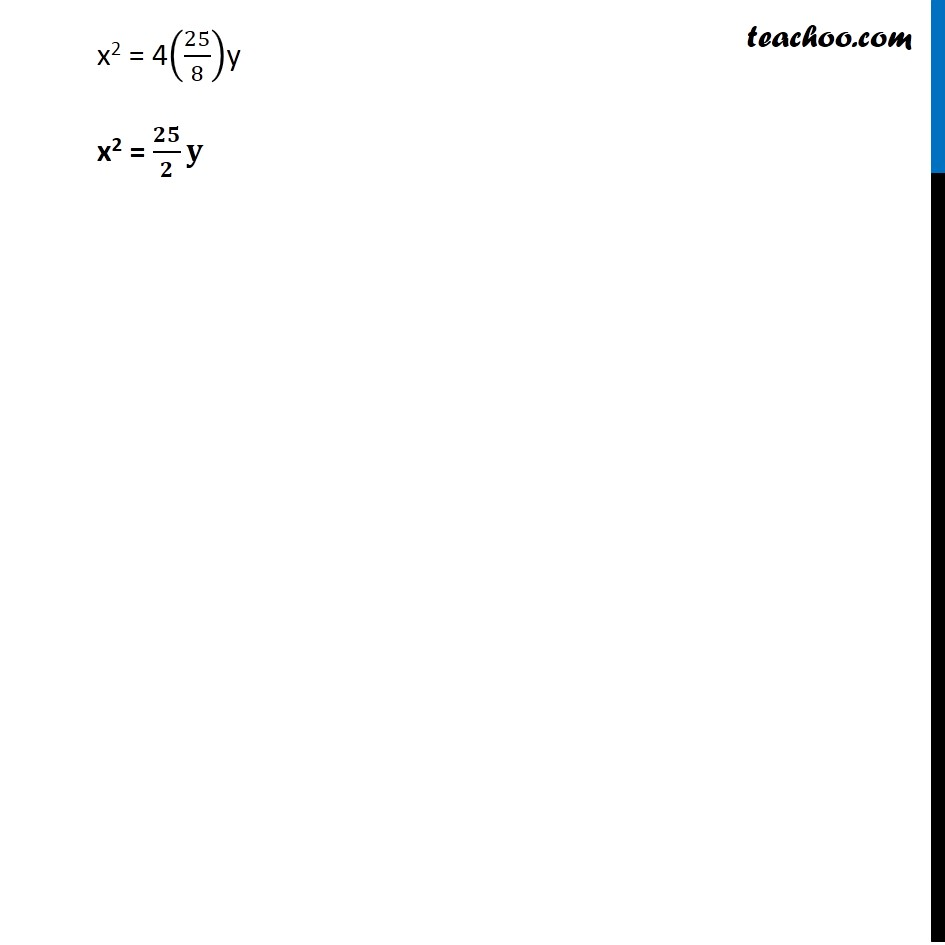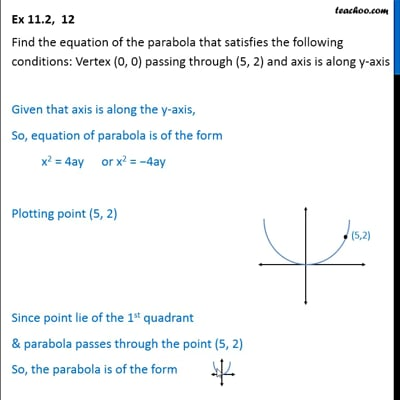Ex 10.2

Chapter 10 Class 11 Conic Sections
Serial order wiseThis video is only available for Teachoo black users

Learn in your speed, with individual attention - Teachoo Maths 1-on-1 Class

### Transcript

Ex 10.2, 12 Find the equation of the parabola that satisfies the following conditions: Vertex (0, 0) passing through (5, 2) and axis is along y-axis Given that axis is along the y-axis, So, equation of parabola is of the form x2 = 4ay or x2 = −4ay Plotting point (5, 2) Since point lie of the 1st quadrant & parabola passes through the point (5, 2) So, the parabola is of the form Hence equation of parabola is x2 = 4ay Point (5, 2) will satisfy the equation of parabola Putting x = 5 & y = 2 in (1) (5)2 = 4a(2) 25 = 8a 8a = 25 a = 𝟐𝟓/𝟖 Hence equation of parabola is x2 = 4ay Putting value of a = 25/8 x2 = 4(25/8)y x2 = 𝟐𝟓/𝟐 𝐲